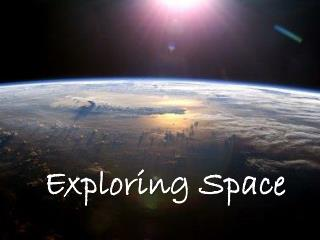DownloadDownload PresentationExploring Space

# Exploring Space

Download Presentation## Exploring Space

- - - - - - - - - - - - - - - - - - - - - - - - - - - E N D - - - - - - - - - - - - - - - - - - - - - - - - - - -
##### Presentation Transcript

1. Exploring Space

2. Radiation From Space • Electromagnetic Spectrum • Energy leaves a star in the form of radiation • Transmitted by electromagnetic waves • Waves arranged according to wavelengths

3. Speed of Light 300,000 km / sec. 186,000 mi / sec. In a vacuum (space)

4. Energy Waves • Crest – Top (highest point) of each wave • Trough – Bottom (lowest point) of a wave • Wavelength – Distance between successive wave crests (troughs) • Frequency – # of crests (troughs) that pass a point in 1 second • Amplitude – distance between a wave’s mid point and its crest (trough)

5. Energy Waves (cont.) • Passage of 1 wave = 1 cycle • 1 cycle / second = 1 hertz (Hz) _________ the wavelength = _________ frequency _________ the wavelength = _________ frequency

6. Energy Waves (cont.) • Passage of 1 wave = 1 cycle • 1 cycle / second = 1 hertz (Hz) The shorter the wavelength = _________ frequency _________ the wavelength = _________ frequency

7. Energy Waves (cont.) • Passage of 1 wave = 1 cycle • 1 cycle / second = 1 hertz (Hz) The shorter the wavelength = Higher frequency _________ the wavelength = _________ frequency

8. Energy Waves (cont.) • Passage of 1 wave = 1 cycle • 1 cycle / second = 1 hertz (Hz) The shorter the wavelength = Higher frequency The longer the wavelength = _________ frequency

9. Energy Waves (cont.) • Passage of 1 wave = 1 cycle • 1 cycle / second = 1 hertz (Hz) The shorter the wavelength = Higher frequency The longer the wavelength = Lower frequency

10. Light • Made up of all colors of the spectrum • Travels in a straight path • Slows down as it travels through different substances

11. R O Y G. B I V

12. RO YG.B I V

13. Light • Reflect – the bouncing of light off the surface of a material • Refract – the bending of light as it passes from one substance to another

14. Prism: angular piece of glass used to produce a spectrum.

15. Colors • White – all colors reflected • Black – all colors absorbed • You don’t “see” black • Sensation when very little light of any color reaches your eye • Green – green, blue, yellow reflected

16. Why do you see the colors that you see?

17. Why do you see the colors that you see?

18. Why do you see the colors that you see?

19. Rainbow Simulator

20. t

21. Telescope

22. Telescope (far.. seeing)

23. Telescope (far.. see) Radio Telescopes Optical Telescopes

24. Telescope (far.. see) Radio Telescopes collects and focuses radio waves Optical Telescopes

25. Telescope (far.. see) Radio Telescopes collects and focuses radio waves Optical Telescopes collects and focuses light rays

26. Telescope (far.. see) Radio Telescopes Optical Telescopes Refracting: uses a lens to bend light to a focal point Reflecting: uses a mirror to bounce light to a focal point

27. Focal Length focal point

28. Focal Length focal point Objective Lens

29. Focal Length Eyepiece focal point Objective Lens

30. Light rays from the eyepiece enter your eye before it crosses the focal plane, therefore the image is not corrected (re-inverted). Eyepiece Objective Lens

31. Focal Length Eyepiece Objective Lens F (objective) Magnification = F (eyepiece)

32. Focal Length Eyepiece Objective Lens 700 mm Magnification = 15 mm

33. Focal Length Eyepiece Objective Lens Magnification = 46.6 x

34. Focal Length Eyepiece Objective Lens 700 mm Magnification = 10 mm

35. Focal Length Eyepiece Objective Lens Magnification = 70 x

36. Focal Length Eyepiece Objective Lens 700 mm Magnification = 5 mm